Spanning Tree and Minimum Spanning Tree

In this tutorial, you will learn about spanning tree and minimum spanning tree with help of examples and figures.

Before we learn about spanning trees, we need to understand two graphs: undirected graphs and connected graphs.

An undirected graph is a graph in which the edges do not point in any direction (ie. the edges are bidirectional).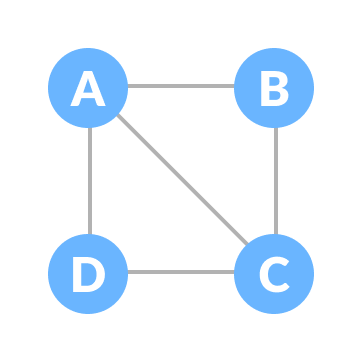A connected graph is a graph in which there is always a path from a vertex to any other vertex.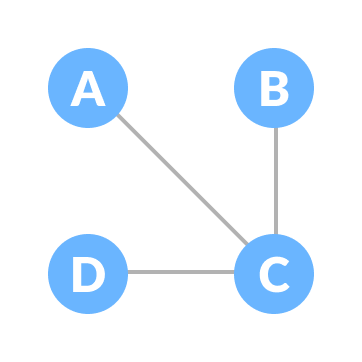Spanning tree

A spanning tree is a sub-graph of an undirected and a connected graph, which includes all the vertices of the graph having a minimum possible number of edges. If a vertex is missed, then it is not a spanning tree.

The edges may or may not have weights assigned to them.

The total number of spanning trees with n vertices that can be created from a complete graph is equal to n(n-2).

Example of a Spanning Tree

Let's understand the spanning tree with examples below:

Let the original graph be: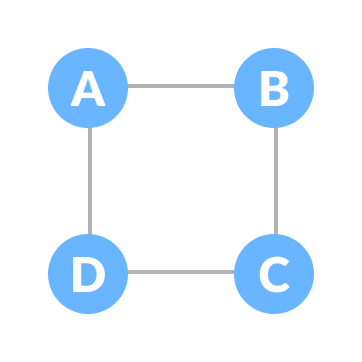Some of the possible spanning trees that can be created from the above graph are: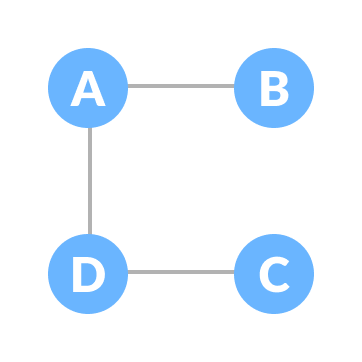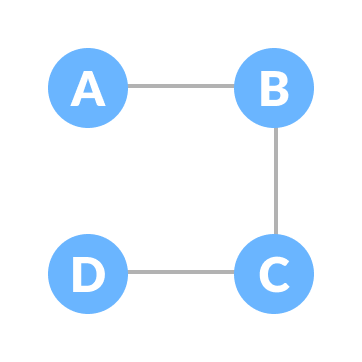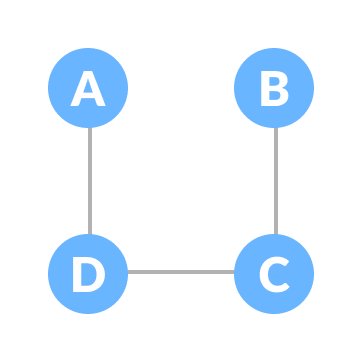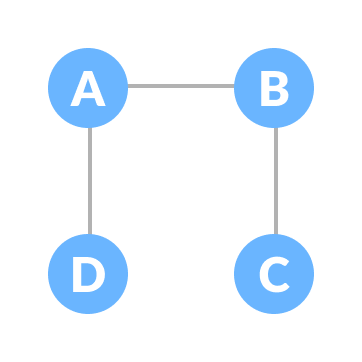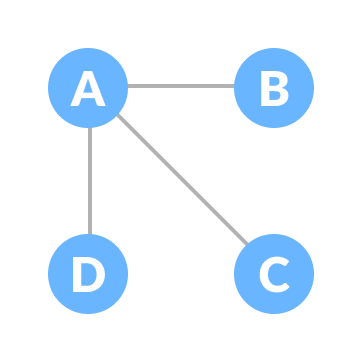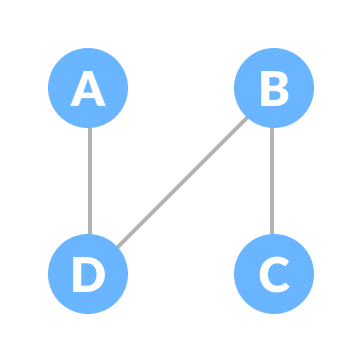We have n = 4, thus the maximum number of possible spanning trees is equal to 44-2 = 16. Thus, 16 spanning trees can be formed from the above graph.

Minimum Spanning Tree

A minimum spanning tree is a spanning tree in which the sum of the weight of the edges is as minimum as possible.

Example of a Spanning Tree

Let's understand the above definition with the help of the example below.

The initial graph is: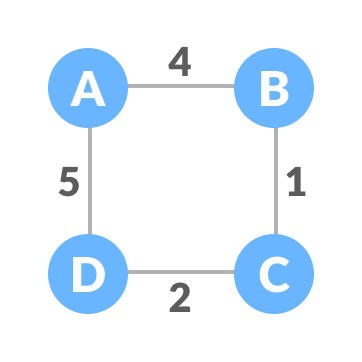The possible spanning trees from the above graph are: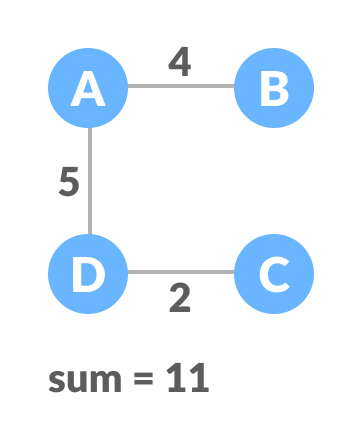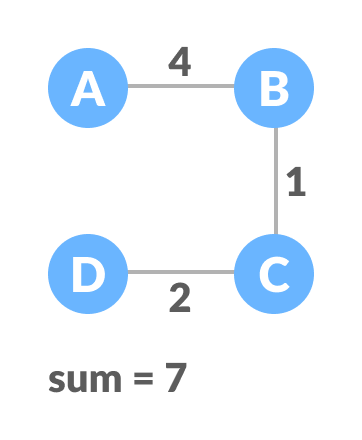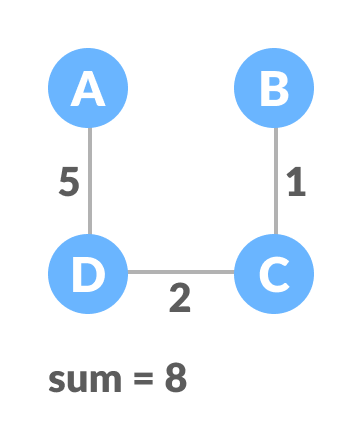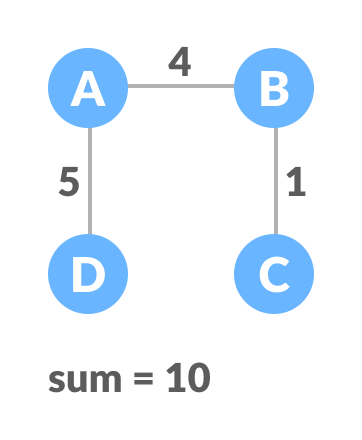The minimum spanning tree from the above spanning trees is:The minimum spanning tree from a graph is found using the following algorithms:

Spanning Tree Applications

• Computer Network Routing Protocol
• Cluster Analysis
• Civil Network Planning

Minimum Spanning tree Applications

• To find paths in the map
• To design networks like telecommunication networks, water supply network and electrical grids.